#### 期刊菜单

Comparative Study of Laser Beam Coherent Combination Technology and Laser Mode-Locking Technology
DOI: 10.12677/OE.2018.84027, PDF , HTML, XML, 下载: 956  浏览: 2,472  国家科技经费支持

Abstract: This paper compared the basic principles of laser beam coherent combination and laser mode-locking, and explained their similarities and differences in nature. It is pointed out that both are technical means to achieve beam energy concentration. The coherent combination of laser beams achieves spatial energy. Concentrated, laser mode-locking achieves energy concentration over time. The laser beam coherent combination is to modulate the transverse mode, and the laser mode-locking is to modulate the longitudinal mode. Technically, both rely on locking the phase of each component to achieve the above purposes, which can be divided into active phase locking and passive phase locking. It also analyzed the possibility that the two technical means can learn from each other.

1. 引言

2. 物理基础

2.1. 相干合成的物理基础

$U\left(x,y\right)=\frac{\mathrm{exp}\left(jkz\right)}{j\lambda z}\mathrm{exp}\left[j\frac{k}{2z}\left({x}^{2}+{y}^{2}\right)\right]ab\text{ }\text{sinc}\left(\frac{ax}{\lambda z}\right)\text{sinc}\left(\frac{by}{\lambda z}\right)$ (1)

$\begin{array}{l}{U}_{1}\left(x,y\right)=\frac{\mathrm{exp}\left(jkz\right)}{j\lambda z}\mathrm{exp}\left[j\frac{k}{2z}\left({x}^{2}+{y}^{2}\right)\right]ab\text{ }\text{sinc}\left(\frac{ax}{\lambda z}\right)\text{sinc}\left(\frac{by}{\lambda z}\right)\\ \text{\hspace{0.17em}}\text{\hspace{0.17em}}\text{\hspace{0.17em}}\text{\hspace{0.17em}}\text{\hspace{0.17em}}\text{\hspace{0.17em}}\text{\hspace{0.17em}}\text{\hspace{0.17em}}\text{\hspace{0.17em}}\text{\hspace{0.17em}}\text{\hspace{0.17em}}\text{\hspace{0.17em}}\text{\hspace{0.17em}}\text{\hspace{0.17em}}\text{\hspace{0.17em}}\text{\hspace{0.17em}}\text{\hspace{0.17em}}\text{\hspace{0.17em}}\text{\hspace{0.17em}}\text{\hspace{0.17em}}\text{\hspace{0.17em}}\text{\hspace{0.17em}}\text{\hspace{0.17em}}\text{\hspace{0.17em}}\text{\hspace{0.17em}}\text{\hspace{0.17em}}\text{\hspace{0.17em}}\text{\hspace{0.17em}}\text{\hspace{0.17em}}\text{\hspace{0.17em}}\text{\hspace{0.17em}}\text{\hspace{0.17em}}\text{\hspace{0.17em}}\text{\hspace{0.17em}}\text{\hspace{0.17em}}\text{\hspace{0.17em}}\text{\hspace{0.17em}}\text{\hspace{0.17em}}\text{\hspace{0.17em}}\text{\hspace{0.17em}}\text{\hspace{0.17em}}\text{\hspace{0.17em}}\text{\hspace{0.17em}}\text{\hspace{0.17em}}\text{\hspace{0.17em}}⋮\\ {U}_{n}\left(x,y\right)=\frac{\mathrm{exp}\left(jkz\right)}{j\lambda z}\mathrm{exp}\left[j\frac{k}{2z}\left({x}^{2}+{y}^{2}\right)\right]ab\text{ }\text{sinc}\left(\frac{ax}{\lambda z}\right)\text{sinc}\left(\frac{by}{\lambda z}\right)\eta \left(\Delta {x}_{n},\Delta {y}_{n}\right)\end{array}$ (2)

$\begin{array}{c}I={|{U}_{1}\left(x,y\right)+\cdots +{U}_{n}\left(x,y\right)|}^{2}\\ =|\frac{\mathrm{exp}\left(jkz\right)}{j\lambda z}\mathrm{exp}\left[j\frac{k}{2z}\left({x}^{2}+{y}^{2}\right)\right]ab\mathrm{sinc}\left(\frac{ax}{\lambda z}\right)\mathrm{sinc}\left(\frac{by}{\lambda z}\right)+\cdots \\ \text{\hspace{0.17em}}\text{\hspace{0.17em}}{+\frac{\mathrm{exp}\left(jkz\right)}{j\lambda z}\mathrm{exp}\left[j\frac{k}{2z}\left({x}^{2}+{y}^{2}\right)\right]ab\mathrm{sinc}\left(\frac{ax}{\lambda z}\right)\mathrm{sinc}\left(\frac{by}{\lambda z}\right)\eta \left(\Delta {x}_{n},\Delta {y}_{n}\right)|}^{2}\\ \text{=}{|\frac{\mathrm{exp}\left(jkz\right)}{j\lambda z}\mathrm{exp}\left[j\frac{k}{2z}\left({x}^{2}+{y}^{2}\right)\right]ab\mathrm{sinc}\left(\frac{ax}{\lambda z}\right)\mathrm{sinc}\left(\frac{by}{\lambda z}\right)\left(1+\underset{i=2}{\overset{n}{\sum }}\eta \left(\Delta {x}_{n},\Delta {y}_{n}\right)\right)|}^{2}\\ ={a}^{2}{b}^{2}{\mathrm{sinc}}^{2}\left(\frac{ax}{\lambda z}\right){\mathrm{sinc}}^{2}\left(\frac{by}{\lambda z}\right){|\left(1+\underset{i=2}{\overset{n}{\sum }}\eta \left(\Delta {x}_{n},\Delta {y}_{n}\right)\right)|}^{2}\end{array}$ (3)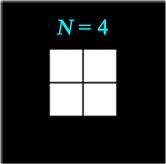(a)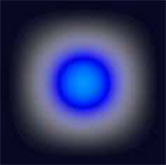(b)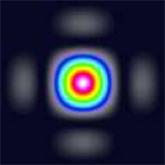(c)

Figure 1. Difference between non-coherent synthesis and coherent synthesis: (a) Near-field arrangement; (b) Non-coherent combined far field; (c) Coherent combined far field

2.2. 激光锁模的物理基础

$E\left(t\right)=A\left(t\right){\text{e}}^{i\left({\omega }_{0}t+{\phi }_{0}\right)}$ (5)

$A\left(t\right)=\frac{{E}_{0}\mathrm{sin}\frac{1}{2}\left(2N+1\right)\left(\Omega t+\beta \right)}{\mathrm{sin}\frac{1}{2}\left(\Omega t+\beta \right)}$ (6)

$I\left(t\right)\propto {A}^{2}\left(t\right)=\frac{{E}_{0}^{2}{\mathrm{sin}}^{2}\frac{1}{2}\left(2N+1\right)\left(\Omega t+\beta \right)}{{\mathrm{sin}}^{2}\frac{1}{2}\left(\Omega t+\beta \right)}$ (7)

$I\propto \left(2N+1\right){E}_{0}^{2}$ (8)

${I}_{m}\propto {E}_{0}^{2}\underset{\left(\Omega t+\beta \right)\to 2m\text{π}}{\mathrm{lim}}\frac{{\mathrm{sin}}^{2}\frac{1}{2}\left(2N+1\right)\left(\Omega t+\beta \right)}{{\mathrm{sin}}^{2}\frac{1}{2}\left(\Omega t+\beta \right)}={\left(2N+1\right)}^{2}{E}_{0}^{2}$ (9)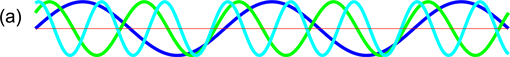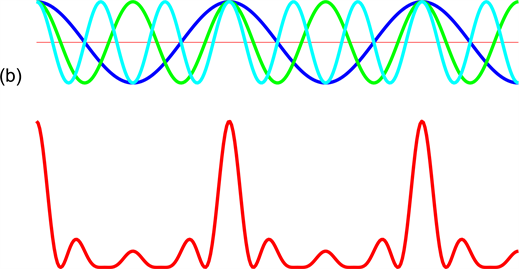Figure 2. (a) Output without mode-lock; (b) Output with mode-lock

2.3. 相干合成与激光锁模的异同

1) 相干合成在空间上实现了能量的汇聚；

2) 相干合成针对同一频率的光束进行相位锁定；

3) 相干合成通常针对谐振腔外的光束进行锁相；

3. 实现相干合成和激光锁模的技术手段分析

3.1. 实现激光光束相干合成的技术手段

1) 被动相干合成通过自组织原理在激光器内部实现多路激光的相位锁定，其实现方式主要有干涉仪方式、非线性自组织方式、倏逝波耦合方式等。往往以复合振荡腔的形式存在。被动式相干合成目前达到的效果不是很好，高能激光系统还比较少见  。

2) 主动相干合成利用一定的控制算法通过相位调制器件在激光器外部实现多路激光的相位锁定，控制方法主要有外差法、自适应锁相法、多抖动法、SPGD算法等  。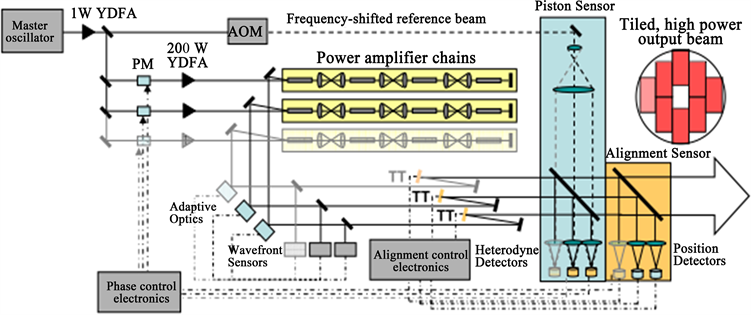Figure 3. Schematic diagram of active-phase-control beam coherent combination by Northrop Grumman Company

3.2. 实现激光锁模的技术手段

1) 主动锁模技术可以分为振幅调制锁模、相位调制锁模以及同步泵浦锁模三种。对于前两种，通常是在腔内置入适当的损耗调制或相位调制元件并控制调制的频率从而实现模式锁定。常用的调制器件是声光和电光损耗调制以及电光相位调制。如果使用一台锁模激光器作为泵浦源，对另一台锁模激光器进行泵浦，通过控制谐振腔内的激光增益，来实现锁模脉冲的输出，这种方法则是同步泵浦锁模方法  。

2) 被动锁模技术。被动锁模技术的种类很多，常用的有自锁模(克尔透镜锁模)、附加脉冲锁模以及可饱和吸收体锁模等。相比于自锁模，附加脉冲锁模技术能够实现自启动，可适用于大多数的固体激光介质  。

4. 技术借鉴的可能性分析

4.1. 将成熟的锁模技术应用到相干合成中的可能性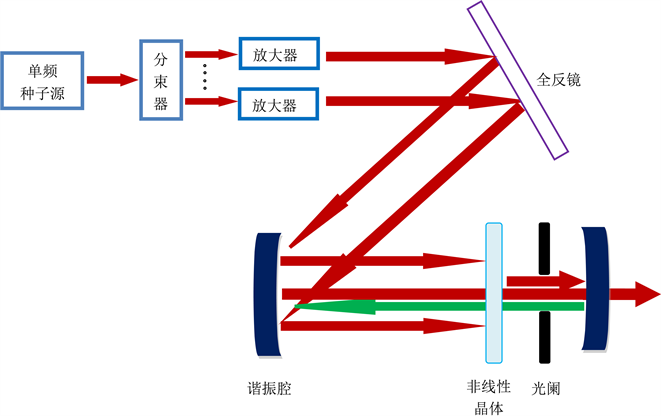Figure 4. Application scheme of typical passive laser mode-locking technology in laser coherent combination technology

4.2. 相干合成技术在锁模中应用的可能性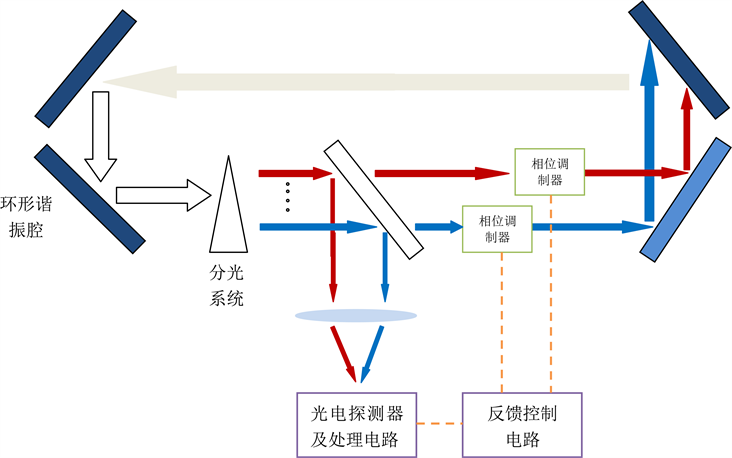Figure 5. Application scheme of typical active phase control coherent combination technology in laser mode-locking technology

1) 激光相干合成的光束在谐振腔外的自由空间，激光锁模针对的光束是在谐振腔内部。若要将成熟的激光锁模技术应用在光束相干合成上面，需要解决如何不在谐振腔里使用被动锁模的技术问题。

2) 激光相干合成的光束在空间上是分离的，激光锁模针对的光束在空间上是纠缠在一起的。若要将成熟的激光相干合成技术应用在激光锁模上面，则需要解决如何分离不同模式的光束，同时加入相位控制单元的问题。

5. 小结

  柏刚, 杨依枫, 晋云霞, 等. 光谱合成激光光束特性的研究进展[J]. 激光与光电子学进展, 2018, 10(30): 1-21.  Tan, D.Z., Sharaflideen, K.N., Yue, Y.Z. and Qiu, J.R. (2016) Femtosecond Laser Induced Phenomena in Transparent Solid Materials: Fundamentals and Applications. Progress in Materials Science, 76, 154-228. https://doi.org/10.1016/j.pmatsci.2015.09.002  Jhon, Y.I., Koo, J., Anasori, B., et al. (2017) Metallic MXene Saturable Absorber for Femtosecond Mode-Locked Lasers. Advanced Materials, 29, 1702496. https://doi.org/10.1002/adma.201702496  苏毅, 万敏. 高能激光系统[M]. 北京: 国防工业出版社, 2004.  Sanchez, T.Y.F.A. () Coherent (Phased Array) and Wavelength (Spectral) Beam Combining Compared. Proceedings Volume 5709, Fiber Lasers II: Technology, Systems, and Applications, San Jose. https://doi.org/10.1117/12.596455  刘敬海. 激光器件与技术[M]. 北京: 北京理工大学出版社, 2004.  周炳琨, 高以智, 陈倜嵘, 等. 激光原理[M]. 第七版. 北京: 清华大学出版社, 2014.  刘泽金, 周朴, 王小林, 等. 激光相干合成的历史、现状与发展趋势[J]. 中国激光, 2010, 37(9): 2221-2234.  郝明明. 大功率半导体激光短阵列合束及光纤耦合技术的研究[D]: [博士学位论文]. 长春: 中国科学院长春光学精密机械与物理研究所, 2012.  Coffey, V. (2014) High-Energy Lasers: New Advances in Defense Applications. Optics and Photonics News, 25, 28-35. https://doi.org/10.1364/OPN.25.10.000028  Zervas, M.N. and Codemard, C.A. (2014) High Power Fiber Lasers: A Review. IEEE Journal of Selected Topics in Quantum Electronics, 20, 219-241. https://doi.org/10.1109/JSTQE.2014.2321279  Goodno, G.D., Komine, H., Mcnaught, S.J., et al. (2006) Coherent Combination of High-Power, Zigzag Slab Lasers. Optics Letters, 31, 1247. https://doi.org/10.1364/OL.31.001247  Kho, J., Prove, R., Harvey, J.D., et al. (2017) 5 nJ, 200 fs, All-Fiber Kaser Mode-Locked with a Nonlinear Amplifying Loop Mirror at 1030 nm. Advanced Solid State Lasers, JTh2A.38.  Chang, M.T., Huang, T.L., Liang, H.C., et al. (2017) Broad Expansion of Optical Frequency Combs by Self-Raman Scattering in Coupled-Cavity Self-Mode-Locked Monolithic Lasers. Optics Express, 25, 7627-7636. https://doi.org/10.1364/OE.25.007627